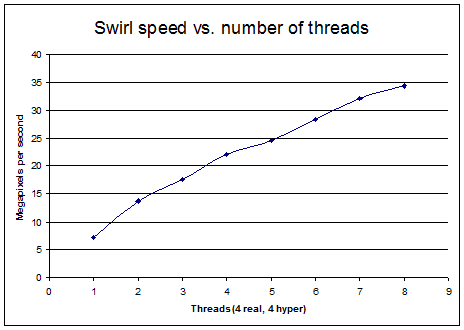## Image twist and swirl algorithm

Pages: 1 2

Be sure to visit the first page if you haven’t already for an explanation of how and why this code works.

```// This code originates from supercomputingblog.com
// You may use this code for any purpose as long as
// credit is properly given to supercomputingblog.com
#define C_PI 3.141592653589793238462643383279502884197169399375

void CImageProcessor::Swirl(Bitmap * pBitmap, double factor)
{

// This function effectively swirls an image

int width = pBitmap->GetWidth();
int height = pBitmap->GetHeight();

double cX = (double)width/2.0f;
double cY = (double)height/2.0f;

BitmapData bitmapData;
pBitmap->LockBits(&Rect(0,0,pBitmap->GetWidth(), pBitmap->GetHeight()), ImageLockModeWrite, PixelFormat32bppARGB, &bitmapData);

unsigned int *pRawBitmapOrig = (unsigned int*)bitmapData.Scan0;   // for easy access and indexing

unsigned int *pBitmapCopy = new unsigned int[bitmapData.Stride*height/4];

memcpy(pBitmapCopy, pRawBitmapOrig, (bitmapData.Stride*height/4) * sizeof(unsigned int));

int nPixels = height*bitmapData.Stride/4;

#pragma omp parallel for
for (int i=0; i < height; i++)
{

double relY = cY-i;
for (int j=0; j < width; j++)
{

double relX = j-cX;
// relX and relY are points in our UV space

// Calculate the angle our points are relative to UV origin. Everything is in radians.
double originalAngle;

if (relX != 0)
{
originalAngle = atan(abs(relY)/abs(relX));

if ( relX > 0 && relY < 0) originalAngle = 2.0f*C_PI - originalAngle;

else if (relX <= 0 && relY >=0) originalAngle = C_PI-originalAngle;

else if (relX <=0 && relY <0) originalAngle += C_PI;
}

else
{
// Take care of rare special case
if (relY >= 0) originalAngle = 0.5f * C_PI;

else originalAngle = 1.5f * C_PI;
}

// Calculate the distance from the center of the UV using pythagorean distance
double radius = sqrt(relX*relX + relY*relY);

// Use any equation we want to determine how much to rotate image by
//double newAngle = originalAngle + factor*radius;	// a progressive twist
double newAngle = originalAngle + 1/(factor*radius+(4.0f/C_PI));

// Transform source UV coordinates back into bitmap coordinates
int srcX = (int)(floor(radius * cos(newAngle)+0.5f));

int srcY = (int)(floor(radius * sin(newAngle)+0.5f));

srcX += cX;
srcY += cY;
srcY = height - srcY;

// Clamp the source to legal image pixel
if (srcX < 0) srcX = 0;

else if (srcX >= width) srcX = width-1;

if (srcY < 0) srcY = 0;

else if (srcY >= height) srcY = height-1;

// Set the pixel color
pRawBitmapOrig[i*bitmapData.Stride/4 + j] = pBitmapCopy[srcY*bitmapData.Stride/4 + srcX];
}
}

delete[] pBitmapCopy;
pBitmap->UnlockBits(&bitmapData);
}```

###Performance

Because this image manipulation requires 64-bit double floating point trigonometry, multiplication and square root, this is really the sort of thing that would fly on any nVidia Tesla compute card. It would also perform very well using high end consumer or Quadro cards. However, since this is a generic image processing tutorial rather than a CUDA tutorial, we’ll just display results using OpenMP instead. The test system is an Intel core-i7 930 at 2.80Ghz with 6GB DDR3 1066 RAM. Even though this CPU only has 4 cores, performance scales surprisingly well to 8 threads due to hyper threading.

Pages: 1 2